• 提示： \begin{align} 与 \begin{equation}不能同时使用 ...这里主要介绍使用\begin{align}的公式编号，以LSTM的公式为例子： 多行公式多编号 % 多行公式多编号 \begin{align} \hat{C}_t &= tanh(W_C\...
目录
多行公式多编号
多行公式单编号，编号居中
多行公式无编号
多行公式多编号，有的行没有编号
一行公式分多行写
情况（case）划分
大括号单编号
大括号多编号

提示：
\begin{align} 与 \begin{equation}不能同时使用\begin{aligned} 只能与 \begin{equation}同时使用要想使用\begin{align}，需要在 \begin{document} 之前插入\usepackage{amsmath}\begin{align}的用法应该与\begin{gather}用法相同，区别是：
\begin{align}是默认右对齐，或者用 & 指定对齐位置\begin{gather} 默认是居中对齐，不能与 & 结合使用
概括：
导言区要加上，amsmath宏包如果想有编号，可以用gather，align如果想某一行没有编号，在用\\换行前使用\nonumber 如果不想有编号，可以gather*，align*，或者在后面加上命令\notag如果想一行公式分开写，用split如果想选择判断，用casesalign，split，cases 的公式对齐，用&

这里主要介绍使用 \begin{align}的公式编号，以LSTM的公式为例子：
多行公式多编号
% 多行公式多编号
\begin{align}
\hat{C}_t &= tanh(W_C\odot[h_{t-1},x_t]+b_C) \\
i_t&=\sigma(W_i\odot[h_{t-1},x_t]+b_i) \\
f_t&=\sigma(W_f\odot[h_{t-1},x_t]+b_f) \\
C_t&=f_t * C_{t-1}+i_t * \hat{C}_t \\
o_t&=\sigma(W_o\odot[h_{t-1},x_t]+b_o) \\
h_t&=o_t * tanh(C_t)
\end{align}
实现效果：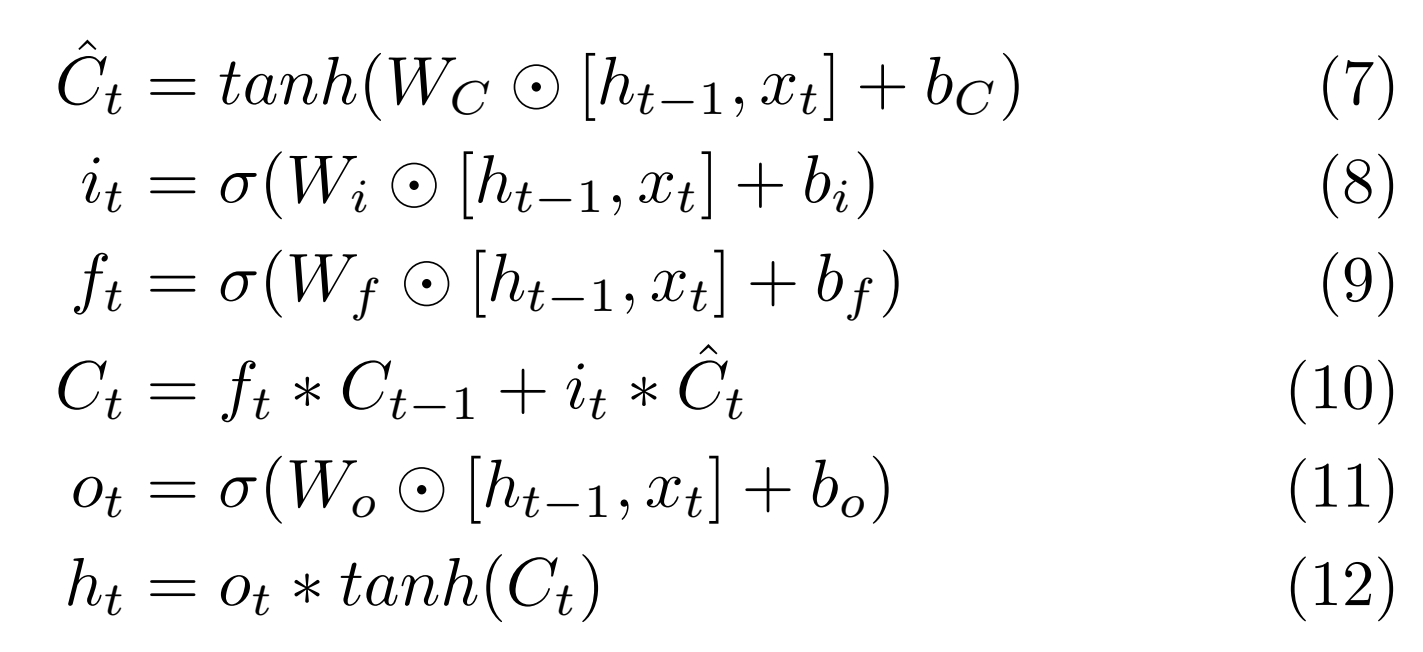多行公式单编号，编号居中
% 多行公式单编号
\begin{equation}
\begin{aligned}
&\hat{C}_t = tanh(W_C\odot[h_{t-1},x_t]+b_C) \\
&i_t=\sigma(W_i\odot[h_{t-1},x_t]+b_i) \\
&f_t=\sigma(W_f\odot[h_{t-1},x_t]+b_f) \\
&C_t=f_t * C_{t-1}+i_t * \hat{C}_t \\
&o_t=\sigma(W_o\odot[h_{t-1},x_t]+b_o) \\
&h_t=o_t * tanh(C_t)
\end{aligned}
\end{equation}
实现效果：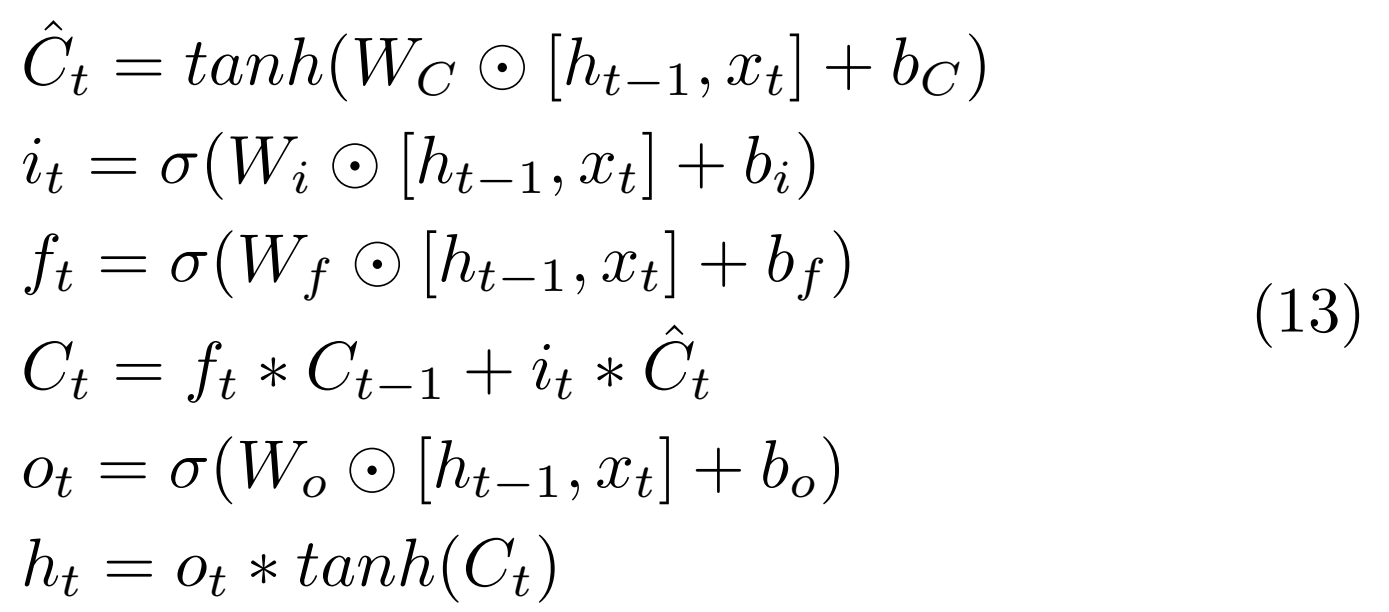多行公式无编号
% 多行公式无编号
\begin{align*}
\hat{C}_t &= tanh(W_C\odot[h_{t-1},x_t]+b_C) \\
i_t&=\sigma(W_i\odot[h_{t-1},x_t]+b_i) \\
f_t&=\sigma(W_f\odot[h_{t-1},x_t]+b_f) \\
C_t&=f_t * C_{t-1}+i_t * \hat{C}_t \\
o_t&=\sigma(W_o\odot[h_{t-1},x_t]+b_o) \\
h_t&=o_t * tanh(C_t)
\end{align*}
实现效果：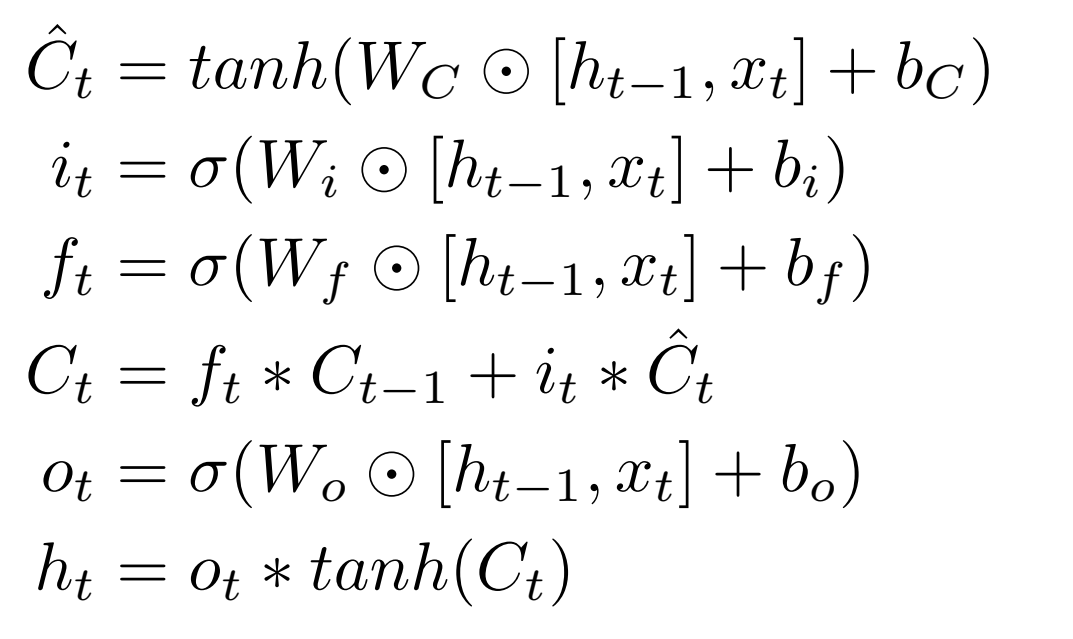多行公式多编号，有的行没有编号
% 多行公式多编号，有的行没有编号
\begin{align}
\hat{C}_t &= tanh(W_C\odot[h_{t-1},x_t]+b_C) \\
i_t&=\sigma(W_i\odot[h_{t-1},x_t]+b_i)  \nonumber \\
f_t&=\sigma(W_f\odot[h_{t-1},x_t]+b_f) \\
C_t&=f_t * C_{t-1}+i_t * \hat{C}_t \\
o_t&=\sigma(W_o\odot[h_{t-1},x_t]+b_o) \\
h_t&=o_t * tanh(C_t)
\end{align}
实现效果：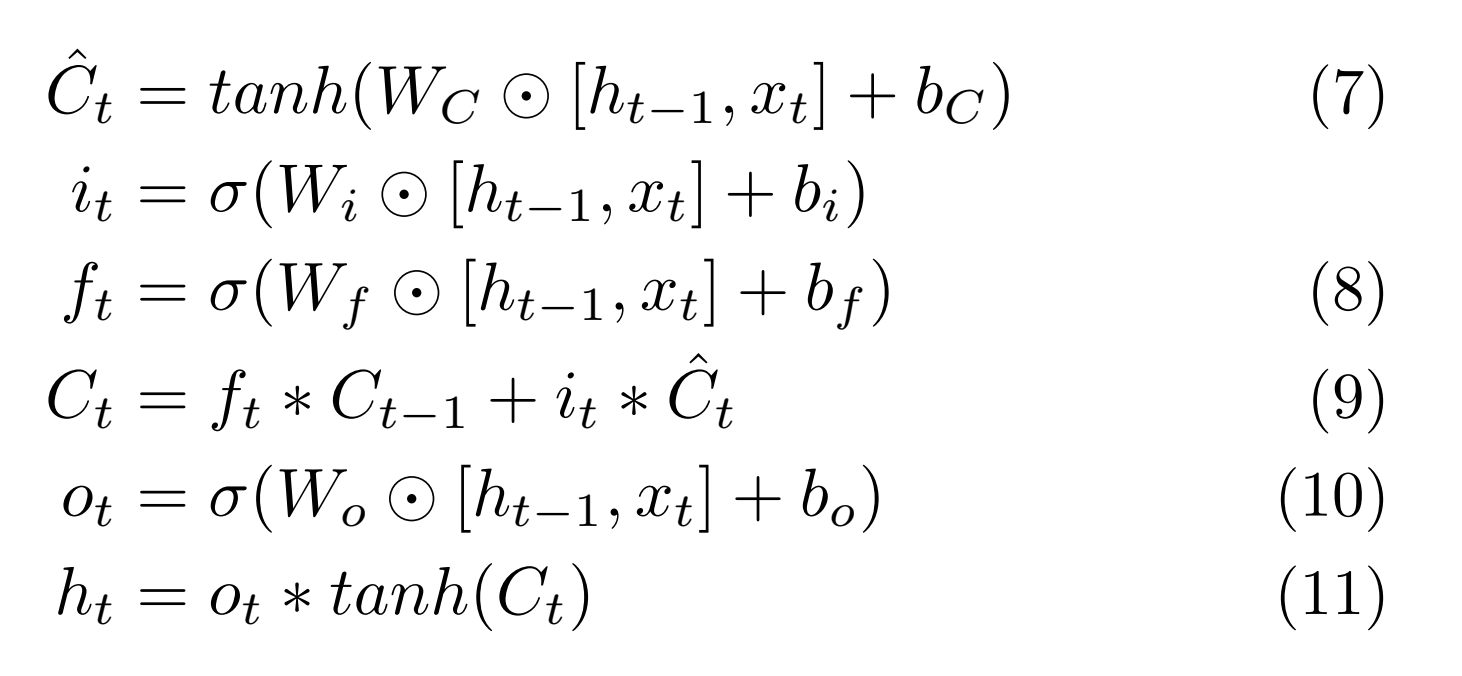一行公式分多行写
%split环境（用对齐）(一个公式分为多行排版) \begin{equation} \begin{split} \cos 2x &= \cos^2 x - \sin^2 x\\ &= 2\cos^2 x - 1 \end{split} \end{equation} 实现效果：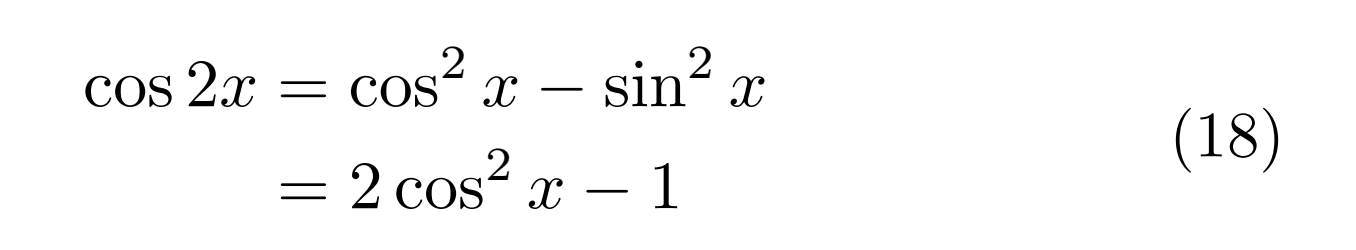情况（case）划分 \begin{equation} D(x) = \begin{cases} 1, & if \ x \in Q \\ 0, & if \ x \in R \end{cases} \end{equation} 实现效果：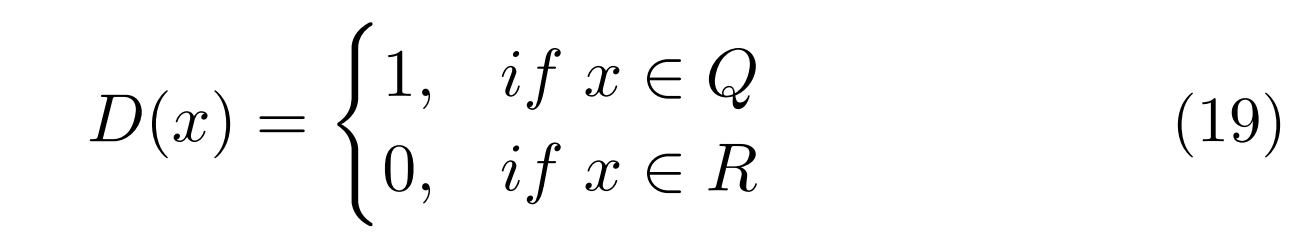大括号单编号 \begin{align} \left\{ %在equation环境下使用，用\left\{命令添加左大括号，用\right.以打点.结束 \begin{aligned} x&=eq1\\ y&=eq2+1 \end{aligned} \right. \end{align} 实现效果：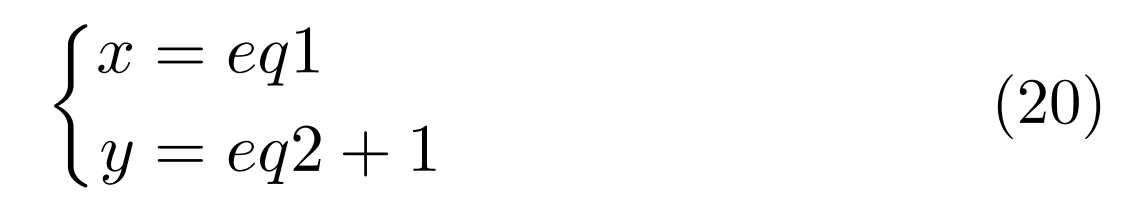大括号多编号 \usepackage{cases} % 大括号多编号 \begin{numcases}{} x_1&=eq1 \label{eqsystem1} \\ x_2+1&=eq2 \label{eqsystem2} \end{numcases} 实现效果：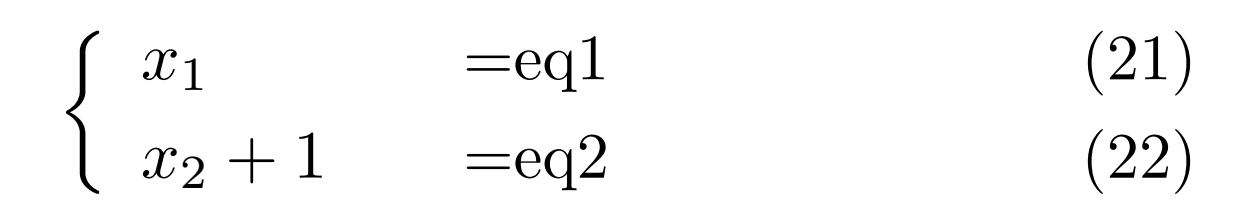更多大括号与编号的情况参考：latex多行公式加大括号、整体编号及多行编号及不同方法的区别 参考：latex12-LaTeX数学公式的多行公式（一）  展开全文• LaTeX数学公式多行公式 %导言区 \documentclass{ctexart} %ctexbook ctexrep %\usepackage{ctex} \usepackage{amsmath} \usepackage{amssymb} %正文区 \begin{document} %可以使用gather环境实现多行公式... LaTeX数学公式的多行公式 %导言区 \documentclass{ctexart} %ctexbook ctexrep %\usepackage{ctex} \usepackage{amsmath} \usepackage{amssymb} %正文区 \begin{document} %可以使用gather环境实现多行公式排版 %内部可以使用\\换行 %带编号 \begin{gather} a + b = b + a \\ ab = ba \end{gather} %不带编号 \begin{gather*} 3 + 5 = 5 + 3 = 8 \\ 3 \times 5 = 5 \times 3 \end{gather*} %在\\前使用\notag 阻止编号 \begin{gather} 3^2 + 4^2 = 5^2 \notag \\ 5^2 + 12^2 = 13^2 \notag \\ a^2 + b^2 = c^2 \end{gather} %align 和 align* 环境 (用&进行对齐 在&位置对齐) %带编号 \begin{align} x &= t + \cos t + 1 \\ y &= 2\sin t \end{align} %不带编号 \begin{align*} %按等号左端对齐 x &= t & x &= \cos t & x &= t \\ 2y &=2t & 2y &= \sin(t+1) & y &= \sin t \end{align*} % split环境 （对齐采用align环境的方式，编号在中间） \begin{equation} \begin{split} \cos 2x &= \cos^2 x - \sin^2 x \\ &= 2\cos^2 x - 1 \end{split} \end{equation} %cases 环境 %每行公式中使用&分隔为两部分， %通常表示值和后面的条件 %\in 表示属于符号 \mathbb用于输出花体字符 需要导入amssymb宏包 %在数学公式中需要使用\text{}临时切换到文本模式 否则无法显示文本 \begin{equation} D(x) = \begin{cases} 1, & \text{如果 } x \in \mathbb{Q}; \\ 0,& \text{如果 } x \in \mathbb{R} \setminus \mathbb{Q}. \end{cases} \end{equation} \end{document} 效果演示：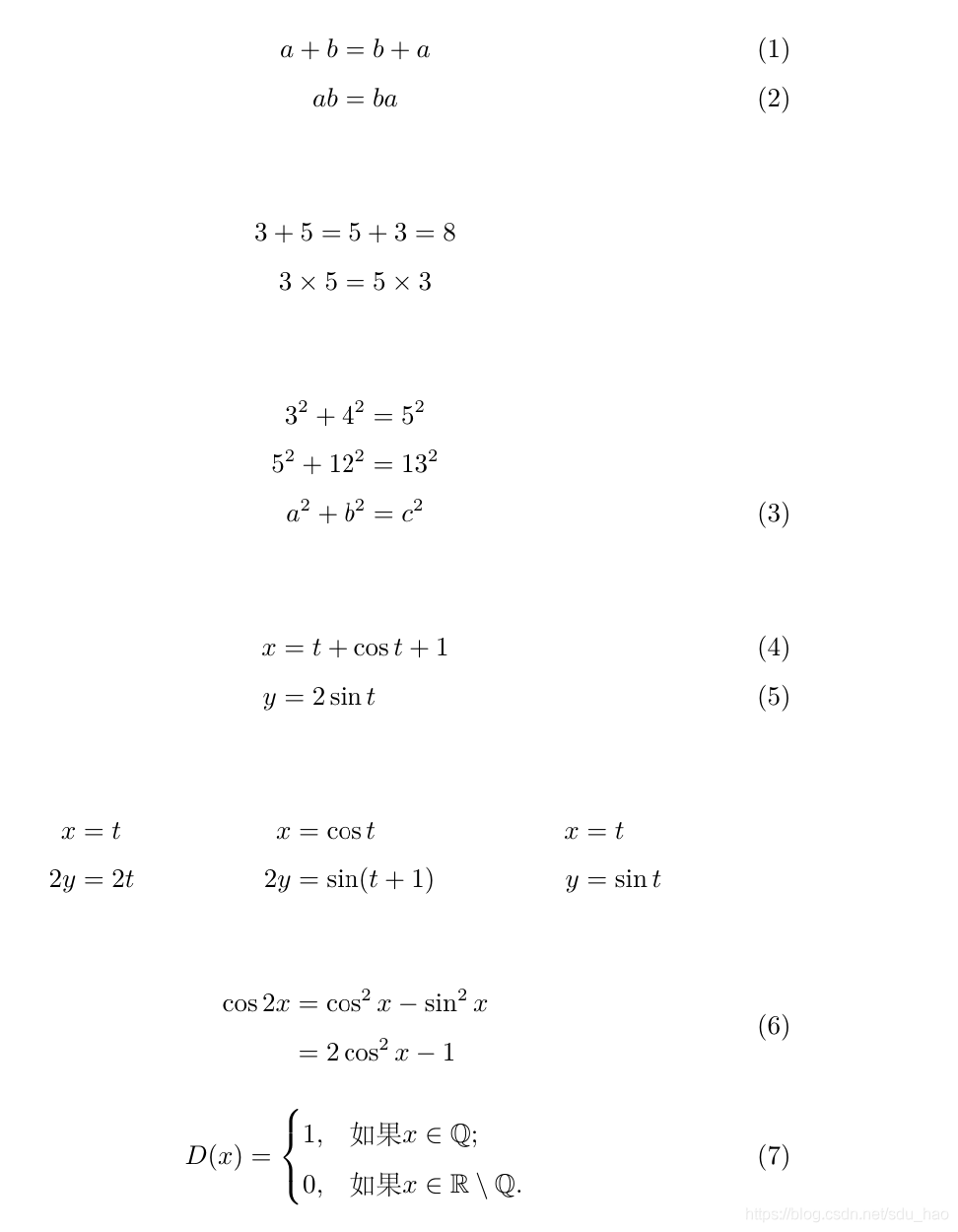展开全文• 如果没有的话，这篇文章的公式类型很多：LaTeX技巧207：使用align环境输入多行公式的技巧 导言区要加上，amsmath宏包 如果想有编号，可以用gather，align 如果不想有编号，可以gather*，align*，或者在后面...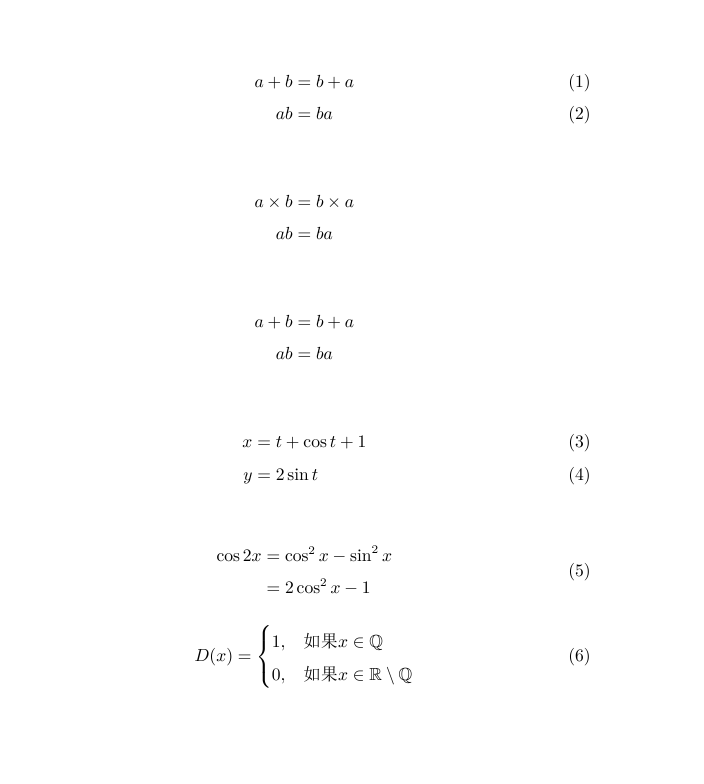上面有些公式的排版，看上那种，直接在下面找代码就好。 如果没有的话，这篇文章的公式类型很多：LaTeX技巧207：使用align环境输入多行公式的技巧 导言区要加上，amsmath宏包 如果想有编号，可以用gather，align 如果不想有编号，可以gather*，align*，或者在后面加上命令\notag 如果想一行公式分开写，用split align和split的公式对其，用
如果想选择判断，用cases
\documentclass{article}

\usepackage{ctex}
\usepackage{amsmath}
\usepackage{amssymb}%花体字符

\begin{document}

\begin{gather}%会产生编号
a+b=b+a\\
ab=ba
\end{gather}

\begin{gather*}%不会产生编号
a \times b=b \times a\\
ab=ba
\end{gather*}

\begin{gather}%会编号
a+b=b+a \notag \\%\notag阻止编号
ab=ba   \notag %\notag阻止编号
\end{gather}

%align和align*环境（用对齐） \begin{align} x &= t + \cos t + 1\\ y &= 2\sin t \end{align} %split环境（用对齐）(一个公式分为多行排版)
\begin{equation}
\begin{split}
\cos 2x &= \cos^2 x - \sin^2 x\\
&= 2\cos^2 x - 1
\end{split}
\end{equation}

%case环境
%每行公式使用&分割成两部分
%通常表示值和后面的条件
\begin{equation}
D(x) = \begin{cases}
1, &\text{如果} x \in \mathbb{Q}\\%mathbb花体字符
0, &\text{如果} x \in \mathbb{R}\setminus\mathbb{Q}
\end{cases}%\text是为了在数学公式中处理中文
\end{equation}

\end{document}


展开全文• 1. 罗列多行公式 2. 拆分单个公式 3. 将公式组合成块 1. 罗列多行公式 基础语法： 1.gather 环境 ：可以在\\ 前使用\notag 命令阻止指定行不编号； \begin{gather} a + b = b + a \\ ...
备注：由于写作环境，内容还未完善！！！ 目录：

文章目录
1. 罗列多行公式2. 拆分单个公式3. 将公式组合成块

1. 罗列多行公式
基础语法：
1.gather 环境 ：可以在\\ 前使用\notag 命令阻止指定行不编号；
\begin{gather}
a + b = b + a \\
ac = ba \notag
\end{gather}

\begin{gather} a + b = b + a \ ac = ba \notag \end{gather}
2.gather* 环境：公式不编号；
\begin{gather*}
a + b = b + a \\
ac = ba
\end{gather*}

\begin{gather*} a + b = b + a \ ac = ba \end{gather*}
3.align 与 align* 环境：（1）允许公式按等号或其他关系符对齐，在关系符前加 &表示对齐；（2）允许排列多列对齐公式，列与列之间使用& 分隔；
\begin{align*}
a &+ b = b + a \\
a &+ c = ba
\end{align*}

\begin{align*} a &+ b = b + a \ a&+c = ba \end{align*}
\begin{align*}
a + b = b + a & a + c = ba & x = \cos t \\
x = y & y = \sin x & y = x + 1
\end{align*}

\begin{align*} a + b &= b + a & a + c &= ba & x &= \cos t \ x &= y & y &= \sin x & y &= x + 1 \end{align*}
（3）特殊对齐需求
\begin{align*}
（a+b）(a^2-ab+b^2)  \\
= a^3 - a^2b + ab^2 + a^2b + ab^2 + b^2   \\
= a^3 + b^3
\end{align*}

\begin{align*} （a+b）(a2-ab+b2) \ = a^3 - a^2b + ab^2 + a^2b + ab^2 + b^2 \ = a^3 + b^3 \end{align*} **注意：**当等式左侧没有公式时，等号与右侧公式间距为0，此时可以使用{}；而且对齐是以符号&为准的；
\begin{align*}
&（a+b）(a^2-ab+b^2)  \\
={} & a^3 - a^2b + ab^2 + a^2b + ab^2 + b^2   \\
&={}  a^3 + b^3
\end{align*}

\begin{align*} &（a+b）(a2-ab+b2) \ ={} & a^3 - a^2b + ab^2 + a^2b + ab^2 + b^2 \ &={} a^3 + b^3 \end{align*}
(4)flalign环境与flalign*环境：与align环境类似，把公式每两列分为一组，分别向右向左对齐，而且公式的间距是可以无限伸长的弹性距离，几列公式会在水平方向分散对齐；  (5)alignat环境与\quad距离调整命令。
2. 拆分单个公式
3. 将公式组合成块
\begin{equation}
D(x) =
\begin{cases}
1, & \text{if } x \in \mathbb{Q	}; \\
0, & \text{if } x \in \mathbb{R}\setminus \mathbb{Q}.
\end{cases}
\end{equation}

\begin{equation} D(x) = \begin{cases} 1, & \text{if } x \in \mathbb{Q }; \ 0, & \text{if } x \in \mathbb{R}\setminus \mathbb{Q}. \end{cases} \end{equation}
\begin{gather} a + b = b + a \ ac = ba \notag \end{gather}
展开全文• %导言区 \documentclass{ctexart} %ctexbook ctexrep %\usepackage{ctex} \usepackage{amsmath} ... %可以使用gather环境实现多行公式排版 %内部可以使用\\换行 %带编号 \begin{gather} a + b =...
• 本文的主要内容有：如何输入行内公式如何输入行间公式多行公式常用的数学符号输入方法相信观察力敏锐的同学一定已经注意到在上一次的推送里的这段代码：\documentclass[a4paper,12pt,landscape,twocolumn]{book}\...
• 3.11 Latex使用笔记 ...多行公式 不需要序号的时候，在使用gather*命令，前后都要改 \begin{gather} \end{gather} 带左弯号的多行公式 \begin{equation} \left\{ %在equation环境下使用 \{ 命令...
• 但是我发现大多数人使用 Mathtype 编辑公式的效率极低，主要体现在不管啥命令都用鼠标在Mathtype 的 UI 界面中选择，这样势必会花费很多时间，因为手一会儿在键盘上，一会儿在鼠标上，很容易分心。...
• 1.自动编号的单行公式环境是 \begin{equation} … \end{equation} 不参与自动编号的单行公式环境： $…$ 人工编号的单行公式可以使用Tex原有的行间公式标记 $$公式 \eqno 编号$$ 将编号放在右边 ...
• from : http://www.boyeut.com/2007/05/equation.html ...1.自动编号的单行公式 ...不参与自动编号的单行公式环境： ...人工编号的单行公式可以使用Tex原有的行间公式标记 ...$$公式 \eqno 编号$$ 将编号放在右边
• 1.自动编号的单行公式环境是 \begin{equation} … \end{equation} 不参与自动编号的单行公式环境： $…$ 人工编号的单行公式可以使用Tex原有的行间公式标记 $$公式 \eqno 编号$$ 将编号放在右边 ...
• ## Latex公式编号问题

万次阅读 多人点赞 2019-12-25 21:37:43
目录对某个公式编号/不编号 在写文章时，我们会遇到各种各样的对公式编号的要求，例如对某些公式标号而对另外一些公式不编号、对某些公式整体编号、对一个拆为几行的较长的公式的最后一行编号等。这篇文章总结了对...
• 1、编号多行的中间 代码：&表示公式对齐，\\是换行 2、每一行都加编号，但是可以选择哪一行不加 代码：包\usepackage{amsmath}，\nonumber加在前面表示该行不加编号 ...
• MathType或者Word中自带公式编辑器是我们的选择，但是MathType和自带公式编辑器中公式符号难以寻找，而且编辑公式时基本都是重复性点击符号，没有常用公式模板。今天，推荐三款公式编辑器，让你编辑公式更加高效快捷...
• 其右端的编号(1)如何显示在两个等式的中间？   利用split环境可以将编号写在中间，如： begin{equation} begin{split} a+b & =1\ c+d & =2 end{split} end{equation}   加入label：   ...
• 目录单独加大括号并自动加整体编号，但不能为每行编号不能加大括号的多行编号带大括号，不会自动编号也不能加编号既要大括号也要多行编号多行编号后缀 单独加大括号并自动加整体编号，但不能为每行编号 \document...
• 基础 首先介绍内联公式和单行公式，内联公式使用单$，单行公式使用双$。 我定义了一个函数： y=axy =axy=...多行公式 推荐使用aligned环境，他还有个兄弟align，基本用法如下 \begin{aligned} f(x) &= ax + b \
• \begin{equation} \label{eqn2} \begin{split} n&=\left[\frac{b-a}{0.01}\right]+1, \\ S&=\frac{1}{n}\sum\limits_{j=1}^{n}(\lambda_{0j}-\lambda_{j}). \end{split} \end{equation}
• 如题，解决LaTeX中的公式跨行左大括号的写法。
• LaTeX数学公式的矩阵、多行公式 目录 矩阵公式 数学公式多行公式 矩阵公式 % 导言区 \documentclass{article} \usepackage{ctex} \usepackage{amsmath} %矩阵宏包 % 创建新命令，自定义省略号类型 \new...
• ## Latex公式及编号

千次阅读 2017-04-07 09:31:14
Latex公式编号 1、latex公式编号 如果用eqnarray环境，只能将编号显示在上面或下面等式的后面，如： \begin{eqnarray} a+b = 1 \\ c+d = 2 \end{eqnarray} 编号显示在第二个等式的后面。   ...
• 将几个公式罗列在一起, 是基本的产生多行公式的方法. latex为此提供了eqnarray和eqnarray环境, 用来输入按等号对其的方程组. 显示方程组的基本输入方法是使用编号的equation环境和不编号equation环境,但是里面的换行...
• Latex多行公式左对齐  (2011-06-27 13:09:59) 转载▼ 标签：  latex   多行公式   左对齐   it 分类： 学习资料 今天想要用latex输入多行公式，参照LATEX一书...
• 所需要的环境还是"align"(或者是align*，不带公式编号)。 语法如下： \begin{align*}\label{2} & X(0) = x(0)W_{N}^{0\cdot0} + x(1)W_{N}^{0\cdot1} + \cdots + x(N-1)W_{N}^{0\cdot(N-1)}\\ & X(1) = x...
• 最近在用Latex写东西，遇到了多行对齐公式的输入与编号问题，尝试了网上的许多方法，最终的解决方法记录如下： 方法一：前边\usepackage{amsmath}，利用equation，内部嵌套split，则会在公式的最后一行进行编号； ...抽象代数
• Latex大括号及多行公式 原创 2013年06月08日 16:36:31 23329 array 中lrc表示各列内容的居左、居中、居右。. [plain] view plain copy \begin{equation}  \...
• 这一讲主要讨论了latex多行数学公式的排版方法。 引入amsmath和amssymb宏包 可以使用 gather 环境实现多行公式的排版。 使用双反斜杠进行换行。 此环境不但实现了对公式的分行排版，并且对每一行公式进行了编号。 ......